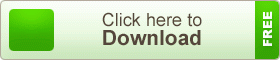# English Version HSC 1st Paper 4th Chapter Physics Note

English Version HSC 1st Paper 4th Chapter Physics Note. Newtonian Mechanics. The physics is one of the oldest academic disciplines, perhaps the oldest through the inclusion of astronomy. In the last two millennia, physics was a part of natural philosophy, chemistry, certain branches of mathematics and biology, but during the scientific revolution in the 17th century, the natural sciences emerged as unique in its own right research programs. Physics intersects with many interdisciplinary areas of research, such as Biophysics and quantum chemistry, and physics limits are not rigidly defined. New ideas in physics often explain the fundamentals of other sciences mechanisms, to open new avenues of research in areas such as mathematics and philosophy.## English Version HSC 1st Paper 4th Chapter Physics Note

Newtonian Mechanics

Question No. I Apu can ride his bicycle around a circular field of radius 20m safely when he is inclined to the center of the field with an angle up to the maximum value of 30°. He was riding at 20kmh-1.

a. What is torque? 1

b. The frictional force is a non-conservative force-Explain. 2

c. How many times are needed to move around the field in crossing the circular path of 5 km? 3

d. Apu will be able to cross the path safely at double speed as mentioned in the stem. Justify. 4

The answer to the question no. 1

a. The vector product of the radius or position vector of a rotating particle and force applied to it is called torque.

b. A force is said to be non-conservative if the work done by the force on a particle in a round trip is not zero. Force of friction is a nonconservative force. We know that frictional force always acts against the motion. Hence in case of a round trip, work done by the frictional force for every segment of the trip is negative. Therefore, for a round trip total work done by this force cannot be zero. Moreover, the work done by frictional force for two fixed points depends on the path followed by the particle. If we push an object on the rough surface of a table from one point to another in different paths, then the work done by the frictional force will certainly be different because of the length of the path i.e., the distance between the two points changes. The magnitude of the work done depends on the path followed. Hence, the frictional force is a non-conservative force.

c. Here given, the radius of circular field r = 20 m.

The circomeference of the circular field is 2 Pai r.

Let, N times are needed to move around the field in crossing the circular the path of 5 km or 5000 m.

.: N= 5000/(3.14×20)

Ans: 40 times

d. The velocity at which the cyclist Apu can turn the corner safety is 20 km h-1 = 5.56 ms-1. If the velocity is made double, Velocity becomes (v) = 2 x 5.56 ms-1 = 11.12 ms-1 Radius of the circular path (r) = 20m

Let, the banking angle for the velocity v is thita.

Thita=32.240.

But Apu can inclined maximum 30° angle when velocity becomes double, banking crosses the maximum limit. So he will not be able to cross the path safely.

Answer to the question no. 2

The practical unit of power before adopting the international system of the unit was horsepower ((H.P) the relation between watt and H.P is 1 H.P = 746 watt.

Elasticity is the property of matter by which object regains original shape and size after removing the deforming force. Intermolecular force is the force of attraction between two molecules. The distance between the molecules. In solid object increases when it is deformed by applying force. Again distance between the molecules in the solid decreases and object regains original shape when the deforming force is removed. Therefore intermolecular force in a solid object is the cause of elasticity

According to the stem, the axis of rotation passes through the midpoint of AB rod. Here the length of the rod AB, l = (1.5 +1.5) m = 3m. Mass of the rod, M = 3 kg, And radius of gyration, k =? According to the condition, k=0.87mPhysics is one of the oldest academic disciplines and, through its inclusion of astronomy, perhaps the oldest. Over the last two millennia, physics, chemistry, biology, and certain branches of mathematics were a part of natural philosophy, but during the scientific revolution in the 17th century, these natural sciences emerged as unique research endeavors in their own right. Physics intersects with many interdisciplinary areas of research, such as biophysics and quantum chemistry, and the boundaries of physics are not rigidly defined. New ideas in physics often explain the fundamental mechanisms studied by other sciences and suggest new avenues of research in academic disciplines such as mathematics and philosophy.

teachingbd24.com is such a website where you will get all kinds of necessary information regarding educational notes, suggestions and question patterns of schools, colleges, and madrasas. Particularly, you will get here special notes of physics that will be immensely useful to both students and teachers. The builder of the website is Mr. Md. Shah Jamal who has been serving for 32 years as an Assistant Professor of Physics at BAF Shaheen College Dhaka. He expects that this website will meet up all the needs of Bengali version learners /students. He has requested concerned students and teachers to spread this website home and abroad.